# How to apply a formula in entire column or row without/with dragging

This post explains that how to apply an excel formula in entire column or row through dragging fill handler in excel. How do I apply formula in entire column or row without dragging fill handler by shortcut or Fill command.

## Apply formula in entire column or row quickly

Assuming that you want to apply a formula to large number of column cells or entire row. You can directly drag the AutoFill handle to all other cells. But if you want to apply a specific formula to hundreds of rows or columns and it should be a very boring or tedious job. So you can use apply formula without dragging by using short cut or Fill Command.

### Dragging AutoFill Handle to apply formula

You can use AutoFill Handle to apply one formula to an entire column or row, assuming that you want to apply one formula to the entire column C, just refer to the follow steps:

1# enter the formula in the first cell in column C.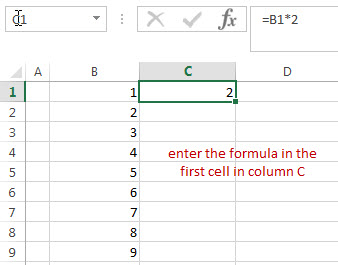2# click “AutoFill Handle” down to the bottom in column C.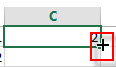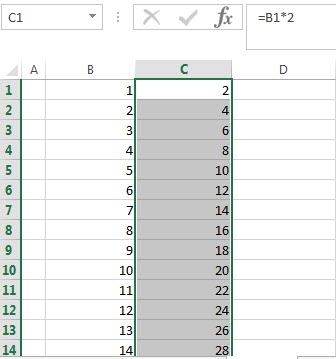You will see that then entire cells in column is applied.

If you want to apply the same formula in the entire row, you just need to drag the AutoFill Handle to right most cell.

### Using shortcut key to apply formula

If you want to apply a formula to the large number of column cells or entire row, you can use the AutoFill Handle, but it is not a good choice. At this moment, you can use shortcut keyboards to apply formula in the entire column or row. Let’s refer to the following steps:

1# click Column C to select the entire Column C.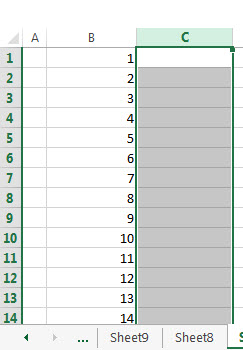2# enter the formula in the formula text box.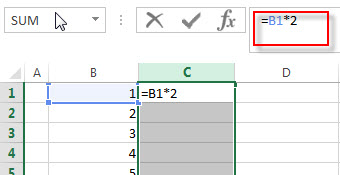3# press “CTRL” +Enter keys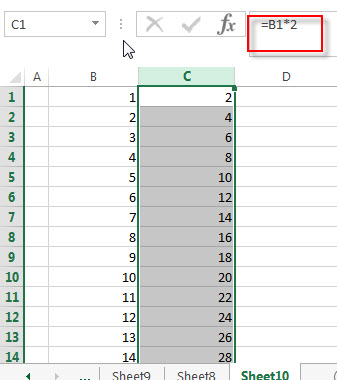You will see that the whole Column C is applied to the same formula.

### Using Fill command to apply formula

You can also use the Fill command to apply a formula in the whole column or row without dragging AutoFill handle. Just refer to the following steps:

1# enter a formula into the first Cell of the Column C (Cell C1).2# select the whole Column C.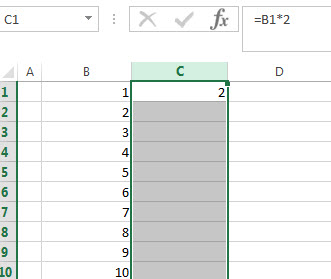3# Click “Down” from the drop down list of “Home”->”Fill” command.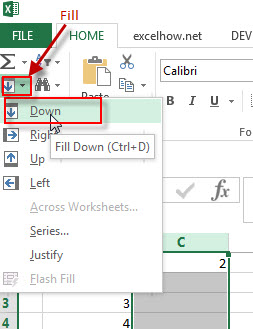If you want to apply the formula to the whole row, you just need to repeat the above steps, just need to select the entire row in the step2. Then click “Right” in the step 3.